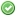rfc:combined-comparison-operator

# PHP RFC: Combined Comparison (Spaceship) Operator

## Introduction

This RFC adds a new operator for combined comparison. Similar to `strcmp()` or `version_compare()` in behavior, but it can be used on all generic PHP values with the same semantics as `<, <=, ==, >=, >`.

## Proposal

Add a new operator `(expr) <=> (expr)`, it returns 0 if both operands are equal, 1 if the left is greater, and -1 if the right is greater. It uses exactly the same comparison rules as used by our existing comparison operators: `<, <=, ==, >=` and `>`. (See the manual for details)

This "three-way comparison operator", also known as the “spaceship operator” (a common name in other languages), works on all standard PHP values. It exists in other languages: Perl, Ruby, and Groovy.

For consistency with Perl, it has the same precedence as `==` and `!=`.

It is implemented by using the result of the existing internal `compare_function` that underlies the other comparison operators. The existing comparison operators could be considered mere shorthands for `<=>`:

operator `<=>` equivalent
`\$a < \$b` `(\$a <=> \$b) === -1`
`\$a <= \$b` `(\$a <=> \$b) === -1 || (\$a <=> \$b) === 0`
`\$a == \$b` `(\$a <=> \$b) === 0`
`\$a != \$b` `(\$a <=> \$b) !== 0`
`\$a >= \$b` `(\$a <=> \$b) === 1 || (\$a <=> \$b) === 0`
`\$a > \$b` `(\$a <=> \$b) === 1`

Here are some examples of its behaviour:

```// Integers
echo 1 <=> 1; // 0
echo 1 <=> 2; // -1
echo 2 <=> 1; // 1

// Floats
echo 1.5 <=> 1.5; // 0
echo 1.5 <=> 2.5; // -1
echo 2.5 <=> 1.5; // 1

// Strings
echo "a" <=> "a"; // 0
echo "a" <=> "b"; // -1
echo "b" <=> "a"; // 1

echo "a" <=> "aa"; // -1
echo "zz" <=> "aa"; // 1

// Arrays
echo [] <=> []; // 0
echo [1, 2, 3] <=> [1, 2, 3]; // 0
echo [1, 2, 3] <=> []; // 1
echo [1, 2, 3] <=> [1, 2, 1]; // 1
echo [1, 2, 3] <=> [1, 2, 4]; // -1

// Objects
\$a = (object) ["a" => "b"];
\$b = (object) ["a" => "b"];
echo \$a <=> \$b; // 0

\$a = (object) ["a" => "b"];
\$b = (object) ["a" => "c"];
echo \$a <=> \$b; // -1

\$a = (object) ["a" => "c"];
\$b = (object) ["a" => "b"];
echo \$a <=> \$b; // 1

\$a = (object) ["a" => "b"];
\$b = (object) ["b" => "b"];
echo \$a <=> \$b; // 0```

Usort Example:

```if ((\$handle = fopen("people.csv", "r")) !== FALSE) {
while ((\$row = fgetcsv(\$handle, 1000, ",")) !== FALSE) {
\$data[] = \$row;
}
fclose(\$handle);
}

// Sort by last name:
usort(\$data, function (\$left, \$right) {
return \$left <=> \$right;
});```

## Usefulness

It makes writing ordering callbacks for use with `usort()` easier. Commonly, users write poor or incorrect ordering functions like this one:

```function order_func(\$a, \$b) {
return \$a >= \$b;
}```

When users do write correct ordering functions, they have to be quite verbose:

```function order_func(\$a, \$b) {
return (\$a < \$b) ? -1 : ((\$a > \$b) ? 1 : 0);
}```

This becomes particularly bad when sorting by multiple columns lexicographically.

With this operator, you can easily write proper ordering functions, like this one:

```function order_func(\$a, \$b) {
return \$a <=> \$b;
}```

Sorting by multiple columns is simpler now, too:

```function order_func(\$a, \$b) {
return [\$a->x, \$a->y, \$a->foo] <=> [\$b->x, \$b->y, \$b->foo];
}```

Or:

```function order_func(\$a, \$b) {
return (\$a->\$x <=> \$b->x) ?: (\$a->y <=> \$b->y) ?: (\$a->foo <=> \$b->foo);
}```

It is also useful in some other contexts.

## Backward Incompatible Changes

This introduces no backwards incompatible changes.

## Proposed PHP Version(s)

The next major version of PHP, currently PHP 7.0.

## New Constants

A `T_SPACESHIP` constant for use with ext/tokenizer has been added.

## Unaffected PHP Functionality

All existing comparison operators are unaffected by this addition.

None.

## Vote

Voting started on 2015-02-02 and will to end on 2015-02-16. As this adds to the PHP language (and hence affects the PHP language specification) a 2/3 majority is required for acceptance. It is a Yes/No vote to accepting the RFC and merging the patch.

Accept the Combined Comparison (Spaceship) Operator RFC and merge patch into master?
Real name Yes No
aharvey (aharvey)ajf (ajf)auroraeosrose (auroraeosrose)brandon (brandon)bwoebi (bwoebi)chregu (chregu)colder (colder)cyberline (cyberline)datibbaw (datibbaw)davey (davey)derick (derick)dm (dm)duodraco (duodraco)fa (fa)francois (francois)guilhermeblanco (guilhermeblanco)gwynne (gwynne)hywan (hywan)irker (irker)jedibc (jedibc)jgmdev (jgmdev)jwage (jwage)kalle (kalle)kinncj (kinncj)klaussilveira (klaussilveira)laruence (laruence)lcobucci (lcobucci)leigh (leigh)levim (levim)lstrojny (lstrojny)malukenho (malukenho)marco (marco)mbeccati (mbeccati)mfischer (mfischer)mfonda (mfonda)mike (mike)mrook (mrook)nikic (nikic)pajoye (pajoye)pauloelr (pauloelr)ramsey (ramsey)rdlowrey (rdlowrey)rdohms (rdohms)salathe (salathe)santiagolizardo (santiagolizardo)seld (seld)sobak (sobak)stas (stas)stelianm (stelianm)thijs (thijs)treffynnon (treffynnon)weierophinney (weierophinney)yohgaki (yohgaki)yunosh (yunosh)Final result: 43 11
This poll has been closed.

## Patches and Tests

A patch targeting php-src master is here: https://github.com/php/php-src/pull/1007

There is not yet a language specification patch.

Shafik's original patch against 5.6 was here: https://github.com/dshafik/php-src/compare/add-spaceship-operator

## Changelog

• v0.2.1 - Clarity on type-juggling behaviour and relation to other comparison operators
• v0.2 - Updated, retargeted to PHP 7 by Andrea
• v0.1 - Initial version by Shafik